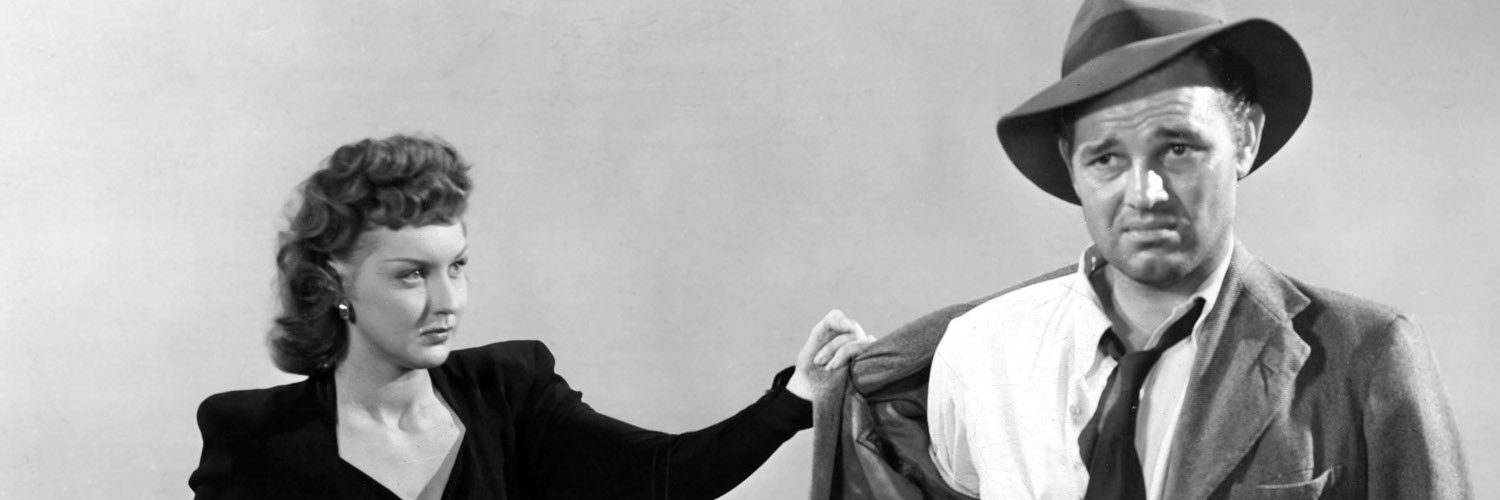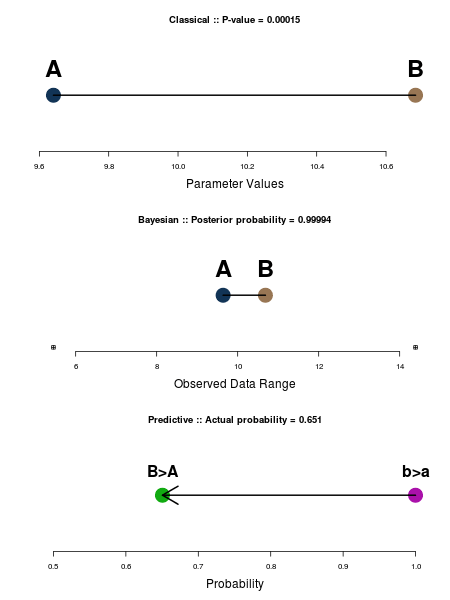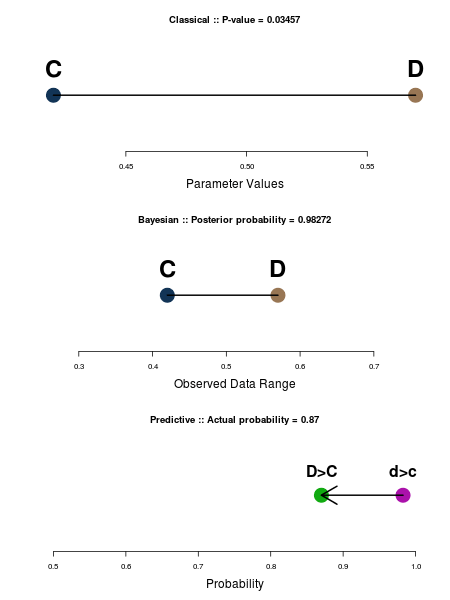Skip to content# Risk Analysis And Over Certainty: Classical vs. Bayesian vs. Predictive StatisticsThere are two main uses of statistics by civilians, defined as folks who use statistics, who may have even had a class or two in the subject, but who are not statisticians1. These two are:

1. Differences between means
2. Differences between proportions

Examples of (1): marketing trial with two groups, A and B; or a drug trial, or psychological, educational, or sociological study, or dozens of other similar academic exercises, and on and on (and on some more). Gist is there are two groups and one wants to test whether the means of these two groups are “different” from one another.

Examples of (2): pretty much the same: marketing trial with two groups, C and D; or a drug trial, or psychological study, etc. Gist is there are two groups and one wants to test whether the proportion of “successes” is “different” from one trial to the other.

There are two main ways data analysis proceeds: the classical, hypothesis-testing, p-value way, or Bayesian posterior examination. Both ways lead to too much certainty. And then there’s the predictive way. Let’s look at some examples.

Differences between means

Two groups, A and B, are observed; data are taken from both. A t-test is run which asks the (unnecessary2) question, “Are the means different?” The first plot below shows us an example: yes, the two means are different, with a p-value of 0.00015. Pretty small! And would lead any researcher to conclude his theory is true. And be pretty darn confident about that judgment.

But he’d be way too certain, as we shall see.Differences between means

A Bayesian would eschew the t-test and opt to tell us of the posterior probability that the parameter for B is larger than the parameter for A. That probability is 0.99994, which is pretty high and allows us to conclude that, yes, the parameter for B is probably larger than the parameter for A. But notice the second plot, which is of the parameters, over the range of the observed data. The difference in the parameters might surely be real (it has a 99.994% chance), but the parameters aren’t the data. They’re just parameters, and are unobservable. The real data is a lot more variable than the Bayesian analysis lets on.

The predictivist goes one step further and says, What do I care about parameters? What’s the chance, given the old observations, that new data for B will be larger than new data for A? That probability is not as high as 0.99994; indeed, it’s much, much smaller. It’s only 0.651. The difference between the Bayesian posterior analysis and the predictive judgment is given in the third plot.

It’s still true that there is a 65% chance that B is bigger than A, but it’s not as sure a thing as the p-value or posterior would have had you believe. This is a much better measure of the inherent uncertainty in the problem. And a much fairer way to look at things.

Differences between proportions

Two groups, C and D, are observed; data are taken from both. A chi-square is run which asks the (unnecessary2) question, “Are the proportions different?” The first plot below shows us an example: yes, the two proportions are different, with a p-value of 0.035. Small. Again, this would lead any researcher to conclude his theory is true. And again be fairly sure about that judgment.

But as before, he’d be too certain.The Bayesian again tells of the posterior distributions, giving us a probability a the parameter for D is larger than C of 0.983. Wow! Those parameters sure have a large chance of being different. But…

Again, the parameters are plotted (second picture) on the range of proportions we’d expect to see if new data were to be taken. Suddenly the difference doesn’t look as big.

The predictivist calculates the probability that in another sample—that is, in new data—the chance there would be more Ds than Cs. That probability is 0.87, which is high, but is not as high as 0.983, and which is what the final picture shows.

There isn’t as much difference between 87% and 98% as in the means test, and maybe big enough not to change any decision you’d make. But it might be enough to change somebody’s decision, and the difference is surely large enough to make it to the bottom line, if these proportions have anything to do with money.

Conclusion

The old ways of looking at things guaranteed over-certainty. The probability one thought there were real differences were always higher—sometimes much higher—than the inherent chance there were differences.

—————————————————————————-

1Note to regular readers: I’m always looking for ways to show the difference between the old and new. Maybe this demonstration fits the bill. I liked it better when it was in my head than on the page. Everything here is purposely telegraphic. I really just want to know if the pictures make sense. This post is really for fellow travelers.

2We know the means, or proportions, are different just by looking. We don’t need statistics to tell of what we already know. Yet this is how most analysis proceeds.

## 8 thoughts on “Risk Analysis And Over Certainty: Classical vs. Bayesian vs. Predictive Statistics” Leave a comment ›

1.Gary says:

As a civilian, let me say the pictures make sense, although the A, B, C, and D parameters look a little too real (like actual data points) when they are constructs to represent distributions. At the risk of cluttering up the pictures, showing the distribution might be a better representation — perhaps as a horizontal bar with density of color (solid at the center, nearly transparent at the tails) depicting the data. This would avoid the usual overlapping bell curves but still convey the idea of distribution.

Now, in civilian terms, how does the predictivist calculate the probability of new data?

2.Ray says:

I’m lost. What is predictive statistics?

3.Ye Olde Statistician says:

I worked in quality control, and one is all-too-aware that if Line 1 uses fresh hydroxide and Line 2 uses recycled hydroxide that any difference between the two lines, no matter how cute the p-value could be due to other factors than the hydroxide that differ between the two lines.

I would also want to know what the predictivist method is. My swag would be to calculate (given a model) the distribution of all possible future

4.Ye Olde Statistician says:

oops.
all possible future results for both and B and figure in what proportion of them B>A. However, in process analysis one is far more likely to encounter situations where the model has changed, so that one is no longer dealing with the same distribution.

5.Rich says:

For maximum marks you should show your working.

6.Doug M says:

Nice tease, what lets see the actual methods employed.

(and links to the nitty-gritty of the calucations would be nice, too)

7.JJD says:

It is nice to know that predictive statistics is superior (with what p value?), but please provide details or links to details explaining how to do it.

8.Chinahand says:

Interesting post – I think I understand what you are doing – though it isn’t as clear as I’d like – it looks like in the first two plots in each example that you are adjusting the scale to account for the spread of the parameters verses the spread of the raw data while the third plot tries to show how much less probable a prediction of future values is compared to the probability one of the underlying parameters is smaller than the other.

Can I ask – what do you mean by prediction – is this predicting whether one new data point from each data set will be greater or lower than the average of its rival data set? Or is it the average of a new sample the same size as previously or what?

I very much agree with the idea of trying to quantify via a prediction, but its important to tell us what the prediction is.

I don’t think you have in this posting, all you’ve said is “new data” – I’m unclear what that exactly means.